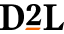# Quiz "Arithmetic Question" and "Multiple Choice" at the same time

How can I have a Quiz type "Arithmetic Question" (algorithmic question) and at the same time "Multiple Choice"?
The "Arithmetic Question" type requires only one answer in the form of a textbox. I would like to have the answers as multiple choice.
Tagged:

•Posts: 21